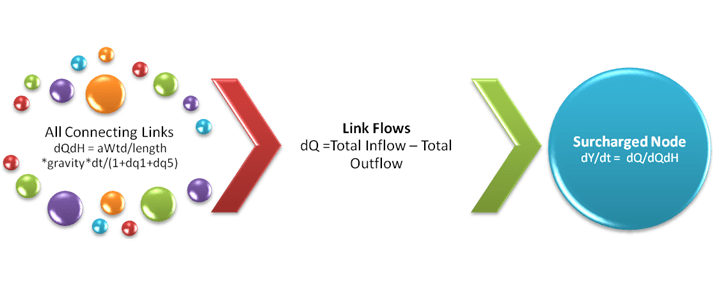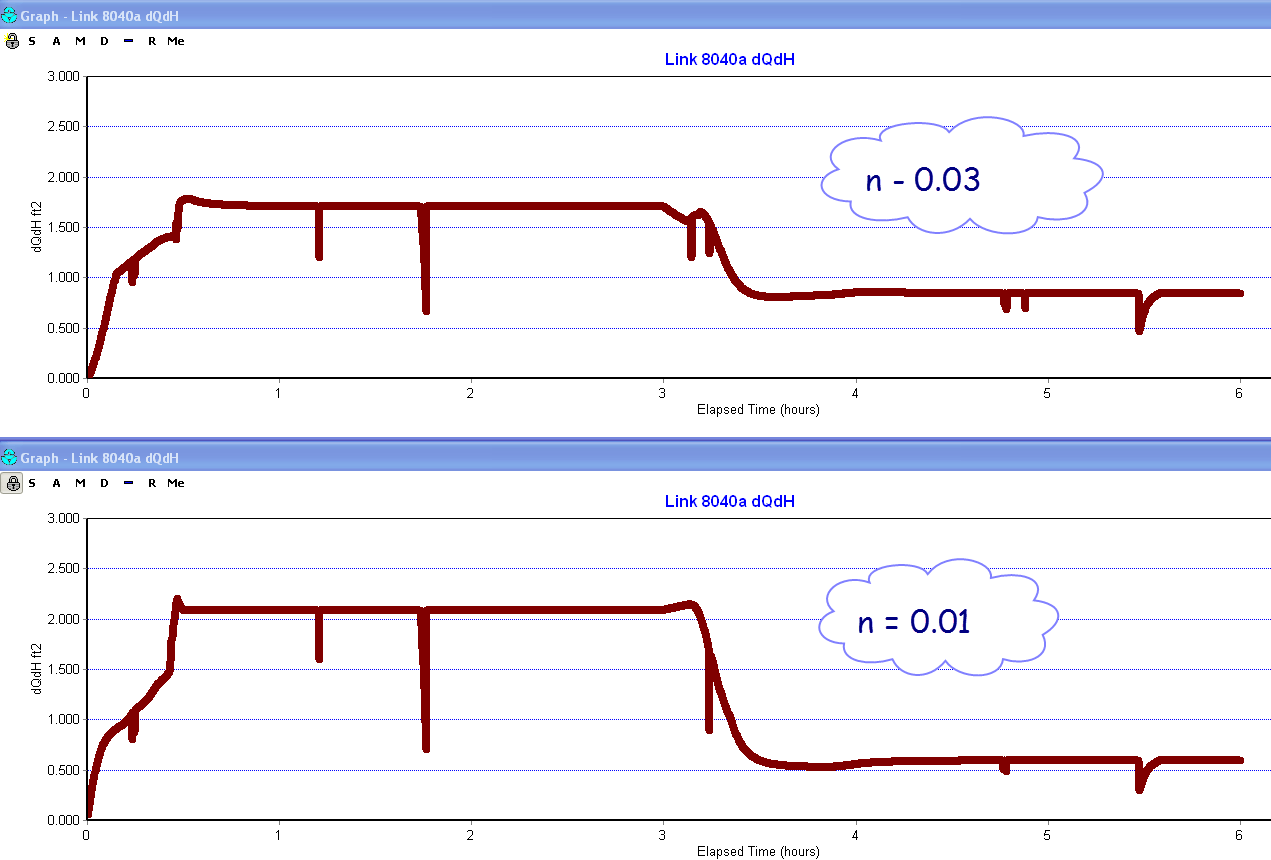#INFOSWMM

# Surcharged Node and the Link Connection in SWMM 5

Subject:   Surcharged Node and the Link Connection in SWMM 5

surcharged node in SWMM 5 uses this point iteration equation (Figure 1):

dY/dt = dQ / The sum of the Connecting Link values of  dQ/dH

where Y is the depth in the node, dt is the time step, H is the head across the link (downstream – upstream), dQ is the net inflow into the node and dQ/dHis the derivative with respect to H of the link  St Venant equation.  If you are trying to calibrate the surcharged node depth, the main calibration variables are the time step and the link  roughness:

1.   Mannings's N

2.   Hazen-Williams or

3.   Darcy-Weisbach

The link roughness is part of the term dq1 in the St Venant solution and the other loss terms are included in the term dq5.  You can adjust the roughness of the surcharged link  to affect the node surcharge depth.

Figure 1.  The Node Surcharge Equation is a function of the net inflow and the sum of the term dQ/dH in all connecting links. Generally, as you increase the roughness the value of dQ/dH increases and the denominator of the term dY/dt = dQ/dQdH increases.Figure 2.  The value of dQ/dH in a link as the roughness of the link increases.